# 强化学习基础知识

## MDP

Posted by MY on July 31, 2020

## MDP

### 马尔可夫过程 Markov Process

#### 马尔可夫性 Markov Property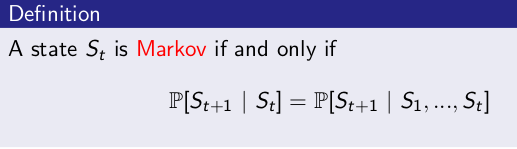• 某一状态信息包含了所有相关的历史。
• 只要当前状态可知，历史信息history就可以被丢弃。
• 当前状态就可以决定未来。The future is independent of the past given the present

$P_{ss’} = P[S_{t+1}=s’|S_t=s]$

$\mathcal{P}=\left[\begin{array}{ccc}{P_{11}} & {\cdots} & {P_{1 n}} \\ {\vdots} & {} & {} \\ {P_{n 1}} & {\cdots} & {P_{n n}}\end{array}\right]$

#### 马尔可夫过程 Markov Process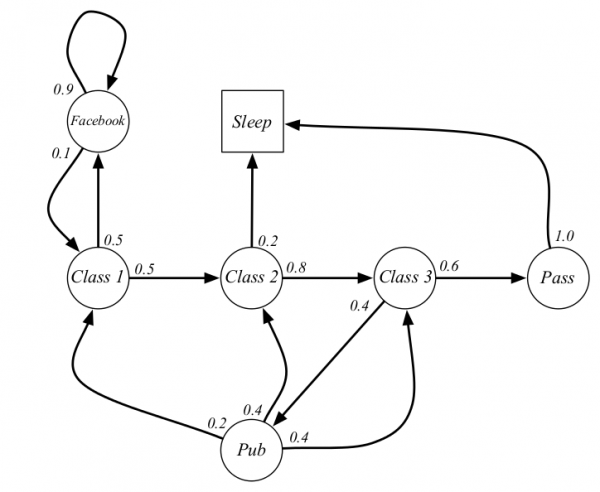### 马尔可夫奖励过程 Markov Reward Process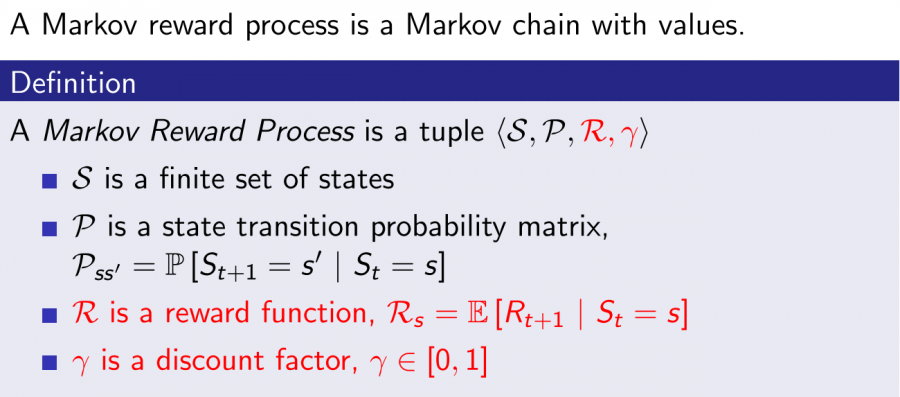• $R$是一个奖励函数。$S$状态下的奖励是某一时刻$t$处在状态$s$下在下一个时刻$t+1$能获得的奖励期望$R_{s} = E[R_{t+1} | S_{t} = s]$
• 衰减系数Discount Factor: $\gamma \in [0, 1]$，其远期利益具有一定的不确定性，符合人类对于眼前利益的追求等。

#### 回报 Return

$G_t = R_{t+1}+\gamma R_{t+2}+…=\sum_{k=0}^{\infty}\gamma^kR_{t+k+1}$

#### 价值函数 Value Function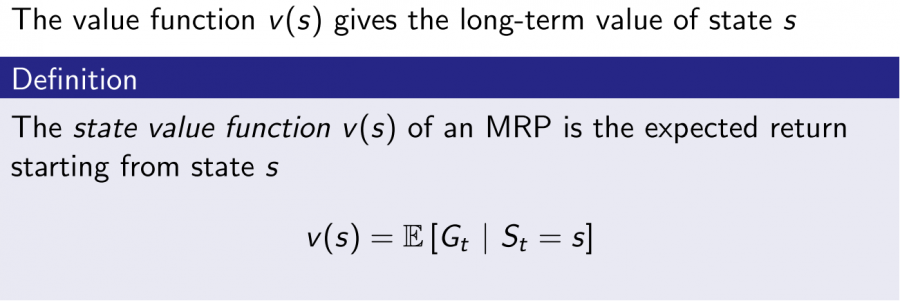状态值函数给出了某一状态或某一动作的长期价值。

#### 价值函数的推导

##### Bellman方程 - MRP

\begin{aligned} v(s) &=\mathbb{E}\left[G_{t} | S_{t}=s\right] \\ &=\mathbb{E}\left[R_{t+1}+\gamma R_{t+2}+\gamma^{2} R_{t+3}+\ldots | S_{t}=s\right] \\ &=\mathbb{E}\left[R_{t+1}+\gamma\left(R_{t+2}+\gamma R_{t+3}+\ldots\right) | S_{t}=s\right] \\ &=\mathbb{E}\left[R_{t+1}+\gamma G_{t+1} | S_{t}=s\right] \\ &=\mathbb{E}\left[R_{t+1}+\gamma v\left(S_{t+1}\right) | S_{t}=s\right] \end{aligned}

\begin{aligned} v(s) &= E[G_t|S_t=s]= E[R_{t+1} + \gamma (R_{t+2}+\gamma R_{t+3}+…) | S_t=s] \\ & = E[R_{t+1} + \gamma G_{t+1} | S_t=s] = R_s + \gamma \sum_{s’\in S}P_{ss’}v(s’) \end{aligned}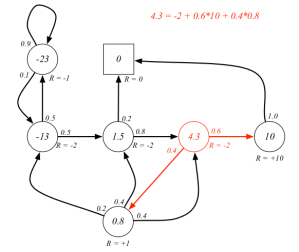##### Bellman方程的矩阵形式和求解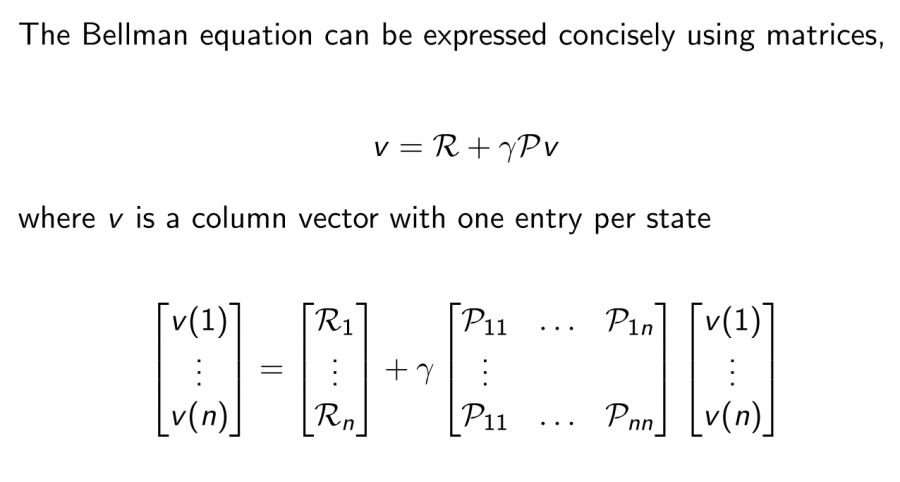### 马尔可夫决策过程 Markov Decision Process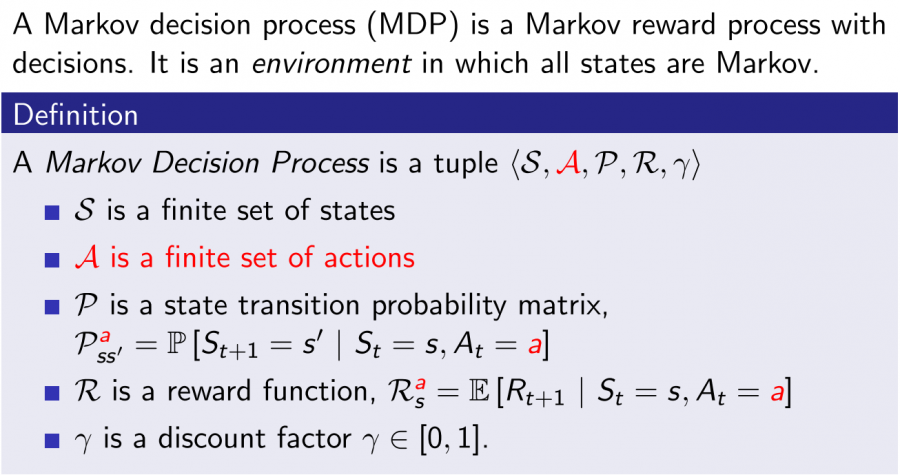$P^a_{ss’} = P[S_{t+1}=s’|S_t=s,A_t=a]$ $R^a_{s} = E[R_{t+1} | S_{t} = s,A_t=a ]$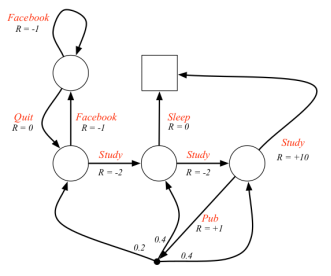#### 基于策略$\pi$的价值函数 Value Function

##### 动作值函数 ACTION-VALUE FUNCTION $Q$

$q_{\pi}(s,a)= E_{\pi}[G_t|S_t=s, A_t=a]$

#### Bellman期望方程 Bellman Expectation Equation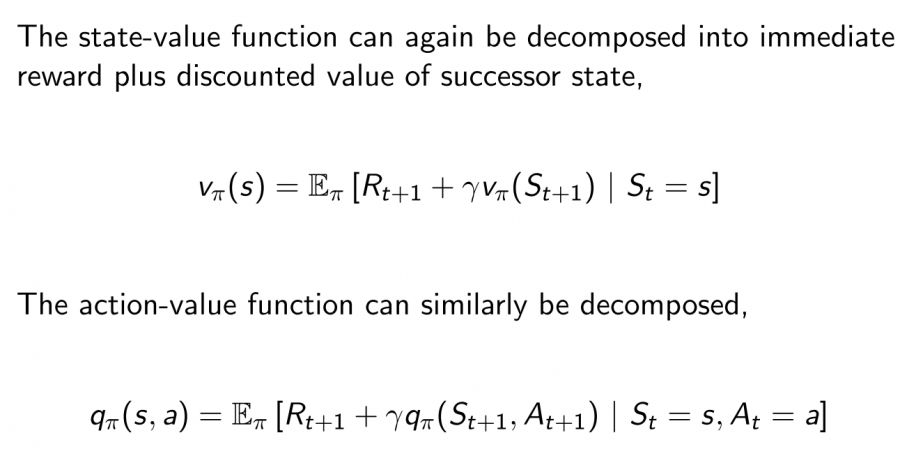• $v_{\pi}(s) = \sum_{a\in A}\pi(a|s)q_{\pi}(s,a)$
• $q_{\pi}(s,a) = R_{s}^{a} + \gamma \sum_{s’\in S}P_{ss’}^a\sum_{a’\in A}\pi(a’|s’)q_{\pi}(s’,a’)$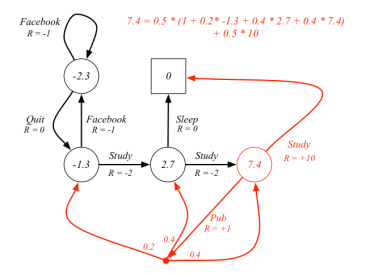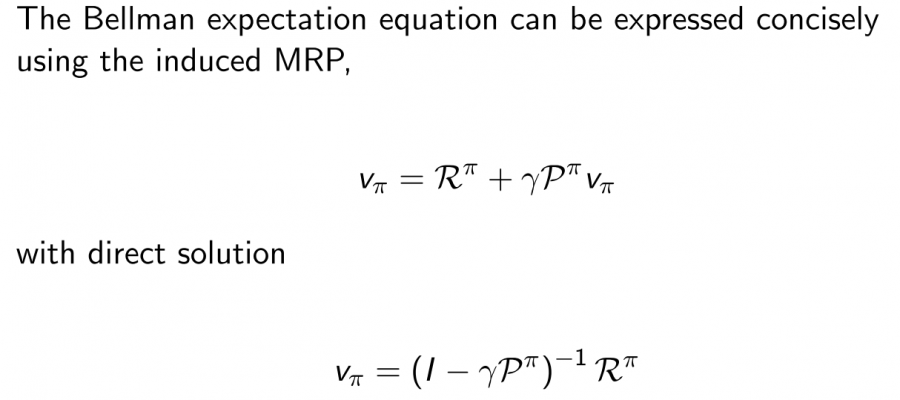#### 最优价值函数

$v_* = \max \limits_{\pi} v_{\pi}(s)$

$q_* (s,a) = \max \limits_{\pi} q_{\pi}(s,a)$

#### 最优策略

$\pi \geq \pi' \quad if \quad v_{\pi}(s)\geq v_{\pi'}(s) ,\forall s$

• 存在一个最优策略，比任何其他策略更好或至少相等。
• 所有的最优策略有相同的最优价值函数。
• 所有的最优策略具有相同的动作值函数。

#### 寻找最优策略

$\pi_{*}(a | s)=\left\{\begin{array}{ll}{1} & {\text { if } a=\underset{a \in \mathcal{A}}{\operatorname{argmax}} q_{*}(s, a)} \\ {0} & {\text { otherwise }}\end{array}\right.$

#### Bellman最优方程 Bellman Optimality Equation

$v_{*}(s)=\max _{a} q_{*}(s, a)$

$q_{*}(s, a)=\mathcal{R}_{s}^{a}+\gamma \sum_{s^{\prime} \in \mathcal{S}} \mathcal{P}_{s s^{\prime}}^{a} v_{*}\left(s^{\prime}\right)$

\begin{aligned} v_{*}(s) &=\max _{a} \mathcal{R}_{s}^{a}+\gamma \sum_{s^{\prime} \in \mathcal{S}} \mathcal{P}_{s s^{\prime}}^{a} v_{*}\left(s^{\prime}\right) \\ q_{*}(s, a) &=\mathcal{R}_{s}^{a}+\gamma \sum_{s^{\prime} \in S} \mathcal{P}_{s s^{\prime}}^{a} \max _{a^{\prime}} q_{*}\left(s^{\prime}, a^{\prime}\right) \end{aligned}

#### 求解Bellman最优方程

Bellman最优方程是非线性的，没有固定的解决方案，通过一些迭代方法来解决：价值迭代、策略迭代、Q-learning、Sarsa等。后续会逐步讲解展开。

##### Share